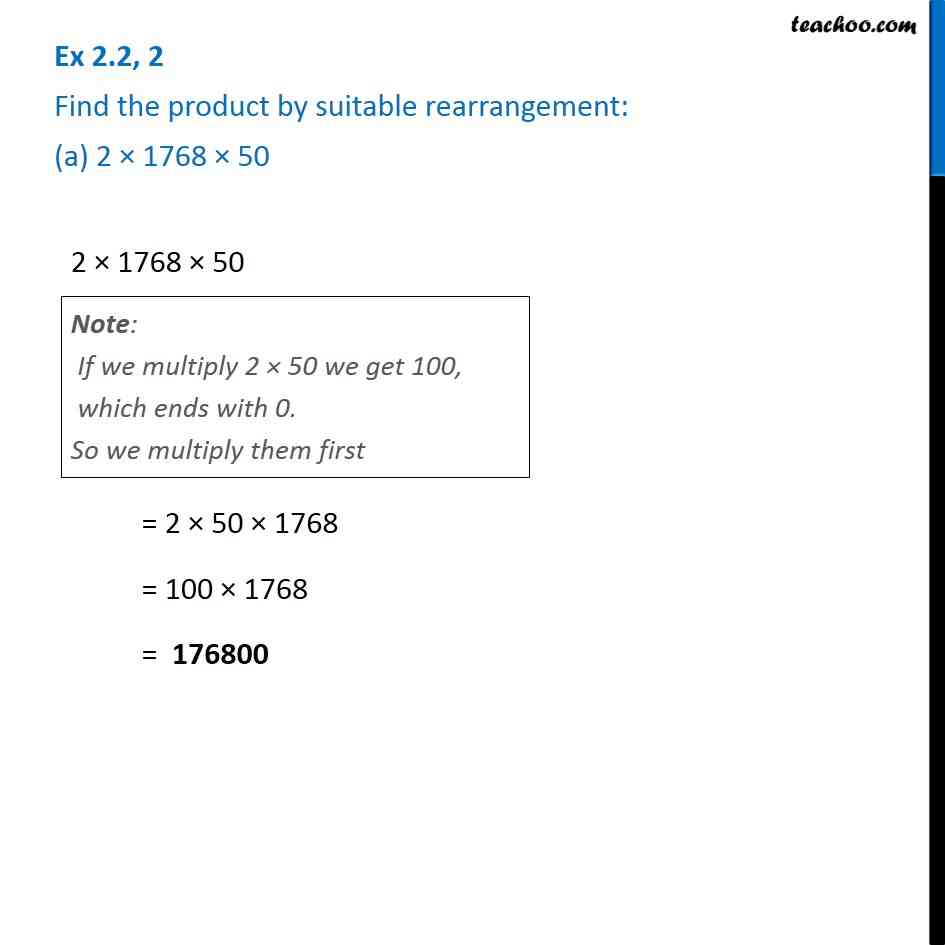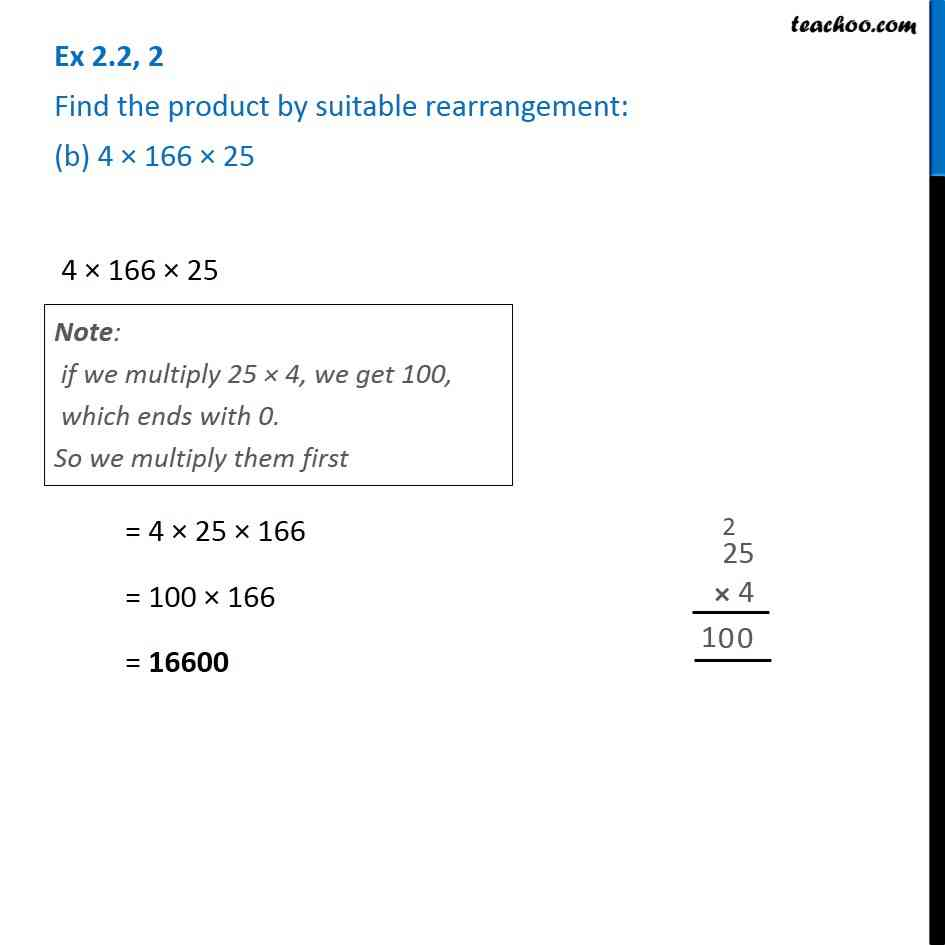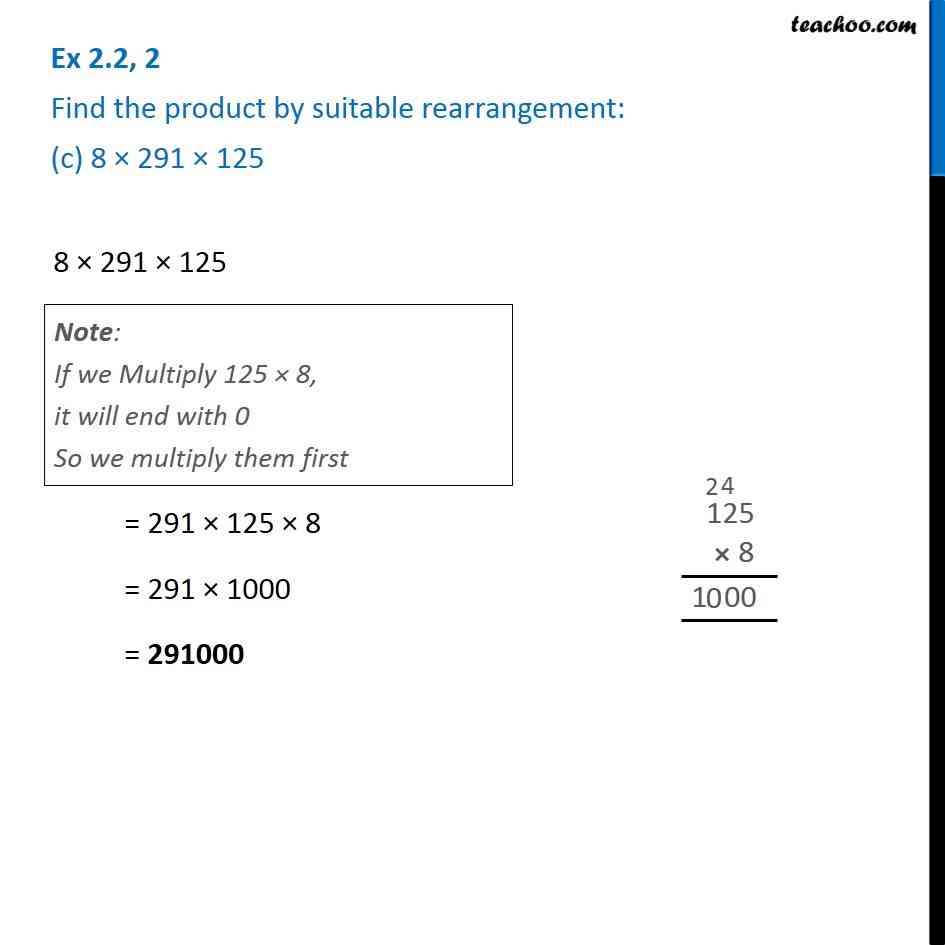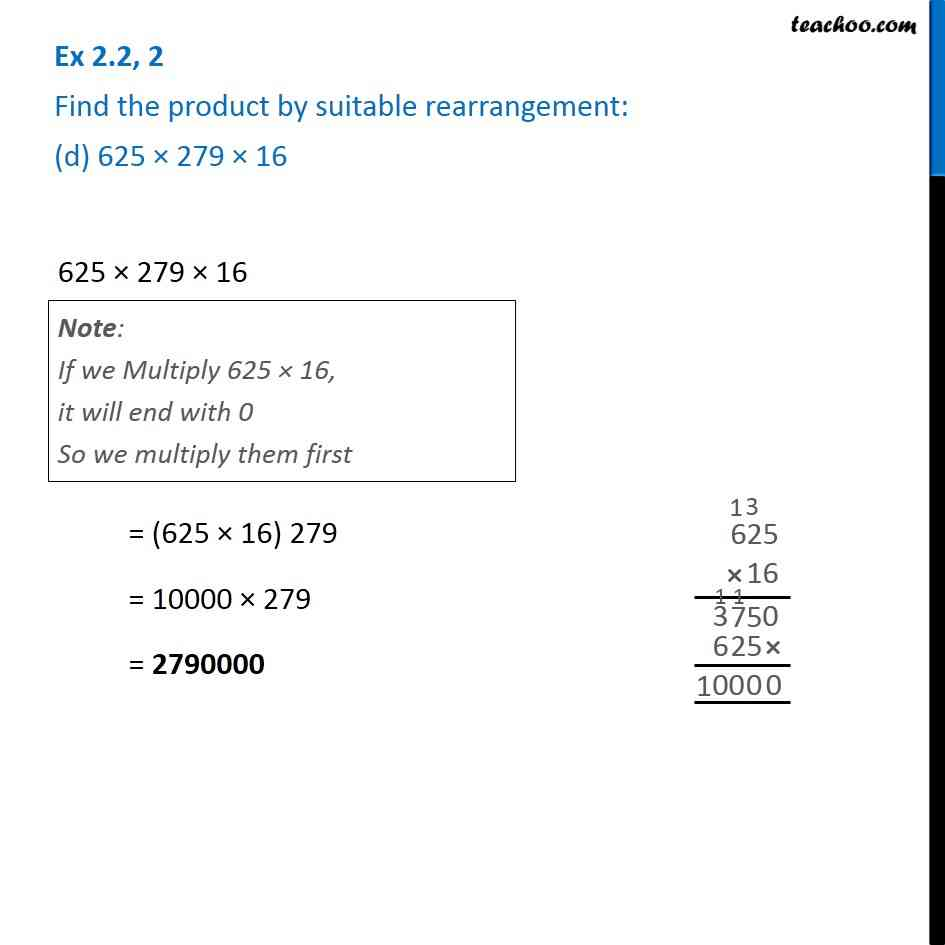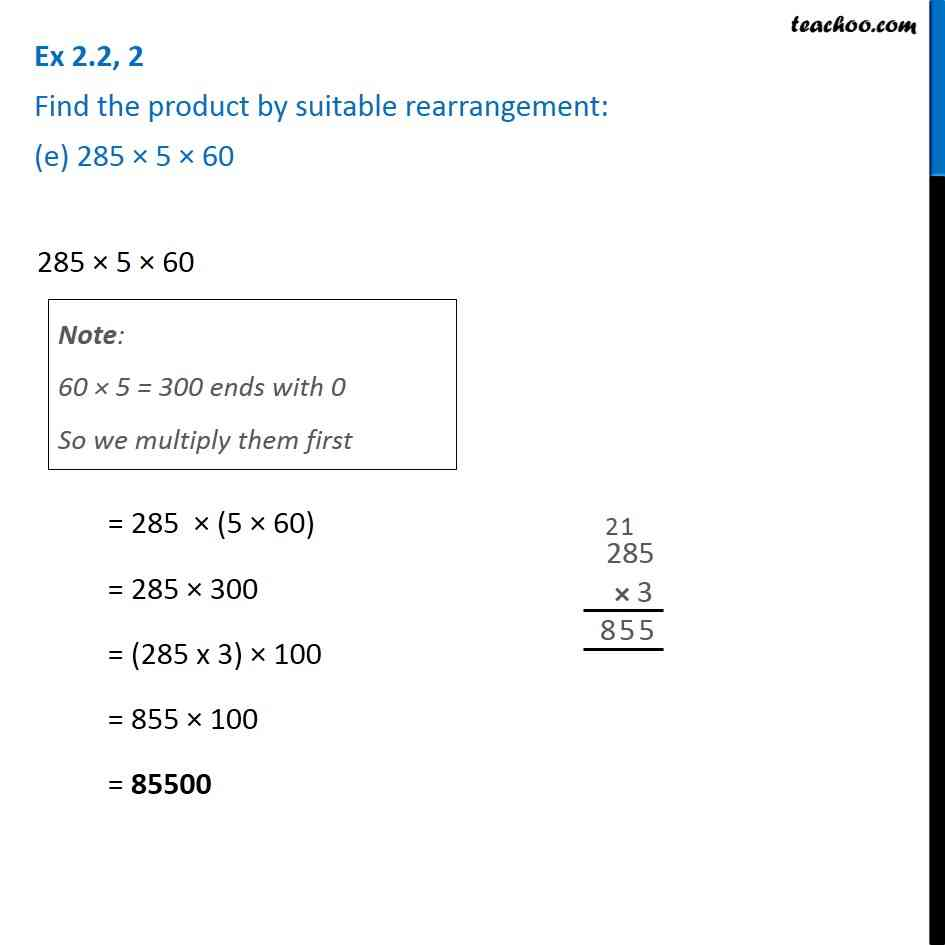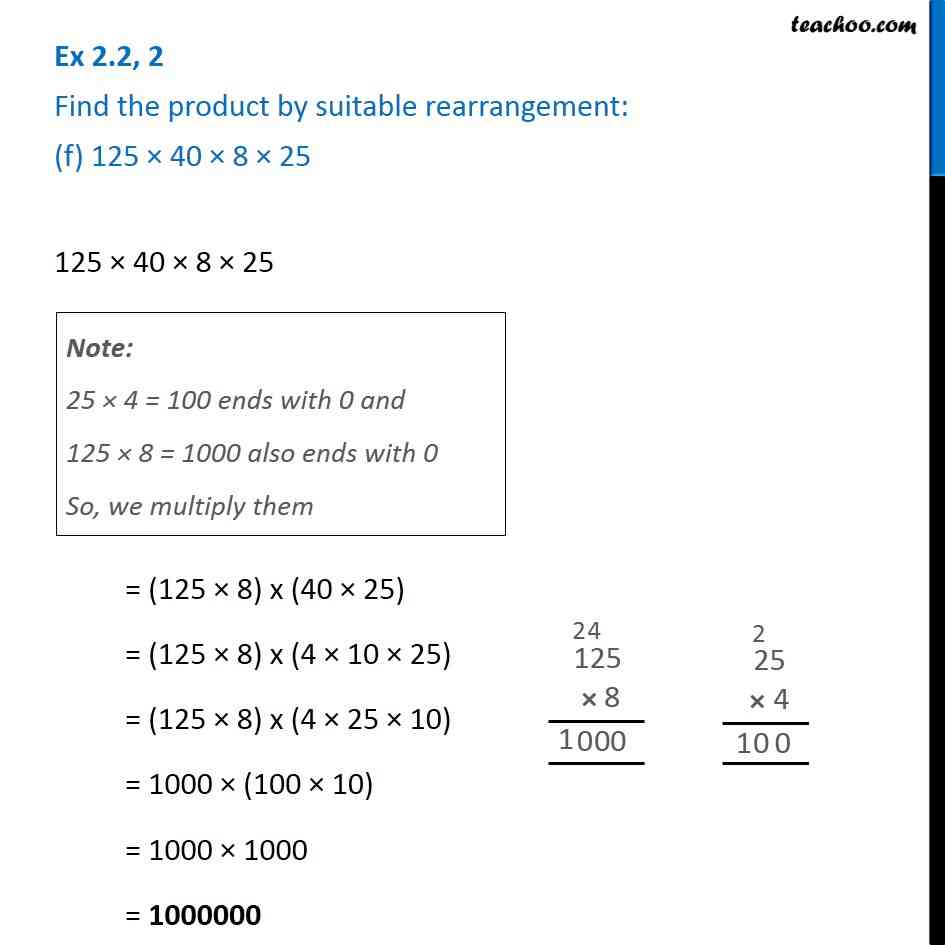1. Chapter 2 Class 6 Whole Numbers
2. Concept wise
3. Multiplication and Addition of Whole numbers

Transcript

Ex 2.2, 2 Find the product by suitable rearrangement: (a) 2 × 1768 × 50 2 × 1768 × 50 = 2 × 50 × 1768 = 100 × 1768 = 176800 Note: If we multiply 2 × 50 we get 100, which ends with 0. So we multiply them first Ex 2.2, 2 Find the product by suitable rearrangement: (b) 4 × 166 × 25 4 × 166 × 25 = 4 × 25 × 166 = 100 × 166 = 16600 Note: if we multiply 25 × 4, we get 100, which ends with 0. So we multiply them first Ex 2.2, 2 Find the product by suitable rearrangement: (c) 8 × 291 × 125 8 × 291 × 125 = 291 × 125 × 8 = 291 × 1000 = 291000 Ex 2.2, 2 Find the product by suitable rearrangement: (d) 625 × 279 × 16 625 × 279 × 16 = (625 × 16) 279 = 10000 × 279 = 2790000 Ex 2.2, 2 Find the product by suitable rearrangement: (e) 285 × 5 × 60 285 × 5 × 60 = 285 × (5 × 60) = 285 × 300 = (285 x 3) × 100 = 855 × 100 = 85500 Ex 2.2, 2 Find the product by suitable rearrangement: (f) 125 × 40 × 8 × 25 125 × 40 × 8 × 25 = (125 × 8) x (40 × 25) = (125 × 8) x (4 × 10 × 25) = (125 × 8) x (4 × 25 × 10) = 1000 × (100 × 10) = 1000 × 1000 = 1000000 Note: 25 × 4 = 100 ends with 0 and 125 × 8 = 1000 also ends with 0 So, we multiply them

Multiplication and Addition of Whole numbers